SNAP Library 6.0, Developer Reference  2020-12-09 16:24:20 SNAP, a general purpose, high performance system for analysis and manipulation of large networks
TBPGraph::TNodeI Class Reference

Node iterator. Only forward iteration (operator++) is supported. More...

`#include <graph.h>`

Collaboration diagram for TBPGraph::TNodeI: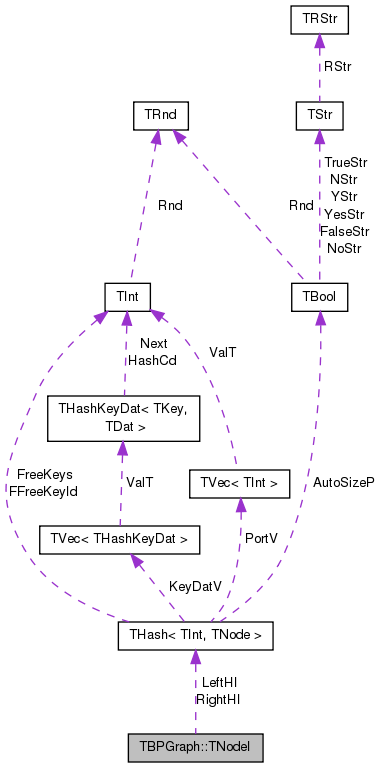[legend]

## Public Member Functions

TNodeI ()

TNodeI (const THashIter &LeftHIter, const THashIter &RightHIter)

TNodeI (const TNodeI &NodeI)

TNodeIoperator= (const TNodeI &NodeI)

TNodeIoperator++ (int)
Increment iterator. More...

bool operator< (const TNodeI &NodeI) const

bool operator== (const TNodeI &NodeI) const

int GetId () const
Returns ID of the current node. More...

bool IsLeft () const
Tests whether the node is left hand side node. More...

bool IsRight () const
Tests whether the node is right hand side node. More...

int GetDeg () const
Returns degree of the current node. More...

int GetInDeg () const
Returns in-degree of the current node (returns same as value GetDeg() since the graph is undirected). More...

int GetOutDeg () const
Returns out-degree of the current node (returns same as value GetDeg() since the graph is undirected). More...

int GetInNId (const int &NodeN) const
Returns ID of NodeN-th in-node (the node pointing to the current node). More...

int GetOutNId (const int &NodeN) const
Returns ID of NodeN-th out-node (the node the current node points to). More...

int GetNbrNId (const int &NodeN) const
Returns ID of NodeN-th neighboring node. More...

bool IsInNId (const int &NId) const
Tests whether node with ID NId points to the current node. More...

bool IsOutNId (const int &NId) const
Tests whether the current node points to node with ID NId. More...

bool IsNbrNId (const int &NId) const
Tests whether node with ID NId is a neighbor of the current node. More...

## Private Types

typedef THash< TInt, TNode >::TIter THashIter

## Private Member Functions

THashIter HI () const

## Private Attributes

THashIter LeftHI

THashIter RightHI

class TBPGraph

## Detailed Description

Node iterator. Only forward iteration (operator++) is supported.

Definition at line 968 of file graph.h.

## Member Typedef Documentation

 typedef THash::TIter TBPGraph::TNodeI::THashIter
private

Definition at line 970 of file graph.h.

## Constructor & Destructor Documentation

 TBPGraph::TNodeI::TNodeI ( )
inline

Definition at line 975 of file graph.h.

975 : LeftHI(), RightHI() { }
THashIter LeftHI
Definition: graph.h:971
THashIter RightHI
Definition: graph.h:971
 TBPGraph::TNodeI::TNodeI ( const THashIter & LeftHIter, const THashIter & RightHIter )
inline

Definition at line 976 of file graph.h.

976 : LeftHI(LeftHIter), RightHI(RightHIter) { }
THashIter LeftHI
Definition: graph.h:971
THashIter RightHI
Definition: graph.h:971
 TBPGraph::TNodeI::TNodeI ( const TNodeI & NodeI )
inline

Definition at line 977 of file graph.h.

977 : LeftHI(NodeI.LeftHI), RightHI(NodeI.RightHI) { }
THashIter LeftHI
Definition: graph.h:971
THashIter RightHI
Definition: graph.h:971

## Member Function Documentation

 int TBPGraph::TNodeI::GetDeg ( ) const
inline

Returns degree of the current node.

Definition at line 995 of file graph.h.

References HI().

995 { return HI().GetDat().GetDeg(); }
THashIter HI() const
Definition: graph.h:973

Here is the call graph for this function: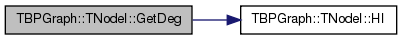int TBPGraph::TNodeI::GetId ( ) const
inline

Returns ID of the current node.

Definition at line 989 of file graph.h.

References HI().

Referenced by TBPGraph::AddNode(), TBPGraph::BegEI(), and TBPGraph::TEdgeI::GetSrcNId().

989 { return HI().GetDat().GetId(); }
THashIter HI() const
Definition: graph.h:973

Here is the call graph for this function: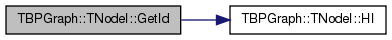Here is the caller graph for this function: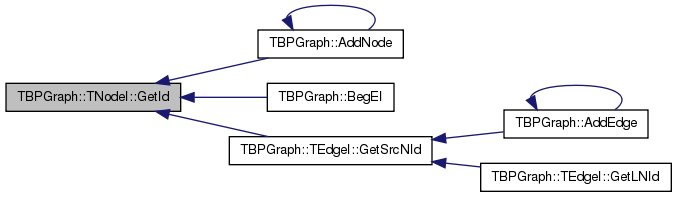int TBPGraph::TNodeI::GetInDeg ( ) const
inline

Returns in-degree of the current node (returns same as value GetDeg() since the graph is undirected).

Definition at line 997 of file graph.h.

References HI().

997 { return HI().GetDat().GetInDeg(); }
THashIter HI() const
Definition: graph.h:973

Here is the call graph for this function: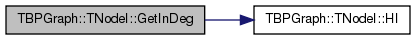int TBPGraph::TNodeI::GetInNId ( const int & NodeN ) const
inline

Returns ID of NodeN-th in-node (the node pointing to the current node).

Definition at line 1002 of file graph.h.

References HI().

1002 { return HI().GetDat().GetInNId(NodeN); }
THashIter HI() const
Definition: graph.h:973

Here is the call graph for this function: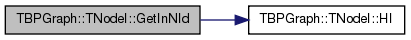int TBPGraph::TNodeI::GetNbrNId ( const int & NodeN ) const
inline

Returns ID of NodeN-th neighboring node.

Definition at line 1008 of file graph.h.

References HI().

1008 { return HI().GetDat().GetNbrNId(NodeN); }
THashIter HI() const
Definition: graph.h:973

Here is the call graph for this function: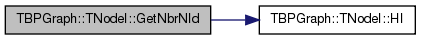int TBPGraph::TNodeI::GetOutDeg ( ) const
inline

Returns out-degree of the current node (returns same as value GetDeg() since the graph is undirected).

Definition at line 999 of file graph.h.

References HI().

Referenced by TBPGraph::BegEI(), and TBPGraph::TEdgeI::operator++().

999 { return HI().GetDat().GetOutDeg(); }
THashIter HI() const
Definition: graph.h:973

Here is the call graph for this function: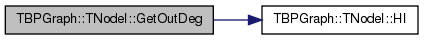Here is the caller graph for this function: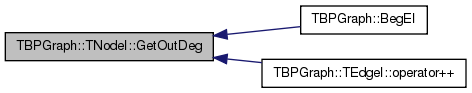int TBPGraph::TNodeI::GetOutNId ( const int & NodeN ) const
inline

Returns ID of NodeN-th out-node (the node the current node points to).

Definition at line 1005 of file graph.h.

References HI().

Referenced by TBPGraph::BegEI(), and TBPGraph::TEdgeI::GetDstNId().

1005 { return HI().GetDat().GetOutNId(NodeN); }
THashIter HI() const
Definition: graph.h:973

Here is the call graph for this function: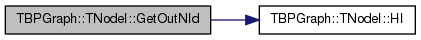Here is the caller graph for this function: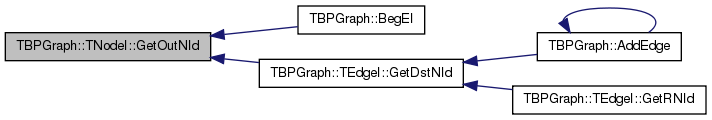THashIter TBPGraph::TNodeI::HI ( ) const
inlineprivate

Definition at line 973 of file graph.h.

References RightHI.

Referenced by GetDeg(), GetId(), GetInDeg(), GetInNId(), GetNbrNId(), GetOutDeg(), GetOutNId(), IsInNId(), IsNbrNId(), and IsOutNId().

973 { return ! LeftHI.IsEnd()?LeftHI:RightHI; }
THashIter LeftHI
Definition: graph.h:971
THashIter RightHI
Definition: graph.h:971

Here is the caller graph for this function: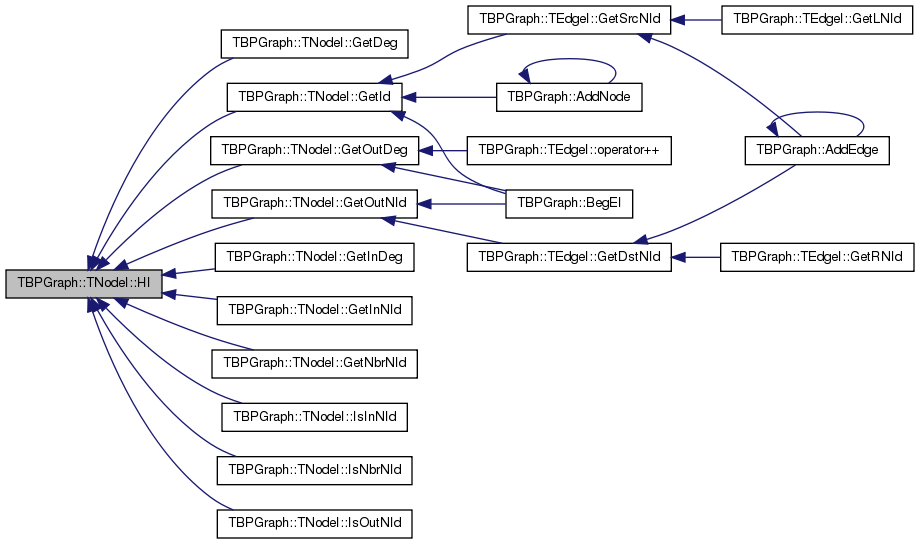bool TBPGraph::TNodeI::IsInNId ( const int & NId ) const
inline

Tests whether node with ID NId points to the current node.

Definition at line 1010 of file graph.h.

References HI().

1010 { return HI().GetDat().IsInNId(NId); }
THashIter HI() const
Definition: graph.h:973

Here is the call graph for this function: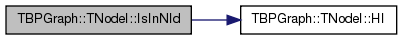bool TBPGraph::TNodeI::IsLeft ( ) const
inline

Tests whether the node is left hand side node.

Definition at line 991 of file graph.h.

991 { return ! LeftHI.IsEnd(); }
THashIter LeftHI
Definition: graph.h:971

Here is the caller graph for this function: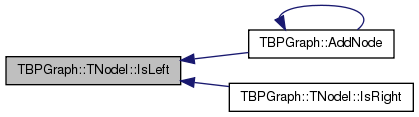bool TBPGraph::TNodeI::IsNbrNId ( const int & NId ) const
inline

Tests whether node with ID NId is a neighbor of the current node.

Definition at line 1014 of file graph.h.

References HI().

1014 { return HI().GetDat().IsNbrNId(NId); }
THashIter HI() const
Definition: graph.h:973

Here is the call graph for this function: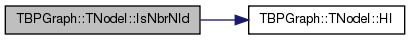bool TBPGraph::TNodeI::IsOutNId ( const int & NId ) const
inline

Tests whether the current node points to node with ID NId.

Definition at line 1012 of file graph.h.

References HI().

1012 { return HI().GetDat().IsOutNId(NId); }
THashIter HI() const
Definition: graph.h:973

Here is the call graph for this function: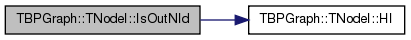bool TBPGraph::TNodeI::IsRight ( ) const
inline

Tests whether the node is right hand side node.

Definition at line 993 of file graph.h.

References IsLeft().

993 { return ! IsLeft(); }
bool IsLeft() const
Tests whether the node is left hand side node.
Definition: graph.h:991

Here is the call graph for this function: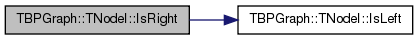TNodeI& TBPGraph::TNodeI::operator++ ( int )
inline

Increment iterator.

Definition at line 980 of file graph.h.

980  {
981  if (! LeftHI.IsEnd()) {
982  LeftHI++; }
983  else if (! RightHI.IsEnd()) {
984  RightHI++; }
985  return *this; }
THashIter LeftHI
Definition: graph.h:971
THashIter RightHI
Definition: graph.h:971
 bool TBPGraph::TNodeI::operator< ( const TNodeI & NodeI ) const
inline

Definition at line 986 of file graph.h.

References LeftHI, and RightHI.

986 { return LeftHI < NodeI.LeftHI || (LeftHI==NodeI.LeftHI && RightHI < NodeI.RightHI); }
THashIter LeftHI
Definition: graph.h:971
THashIter RightHI
Definition: graph.h:971
 TNodeI& TBPGraph::TNodeI::operator= ( const TNodeI & NodeI )
inline

Definition at line 978 of file graph.h.

References LeftHI, and RightHI.

978 { LeftHI = NodeI.LeftHI; RightHI=NodeI.RightHI; return *this; }
THashIter LeftHI
Definition: graph.h:971
THashIter RightHI
Definition: graph.h:971
 bool TBPGraph::TNodeI::operator== ( const TNodeI & NodeI ) const
inline

Definition at line 987 of file graph.h.

References LeftHI, and RightHI.

987 { return LeftHI==NodeI.LeftHI && RightHI==NodeI.RightHI; }
THashIter LeftHI
Definition: graph.h:971
THashIter RightHI
Definition: graph.h:971

## Friends And Related Function Documentation

 friend class TBPGraph
friend

Definition at line 1015 of file graph.h.

## Member Data Documentation

 THashIter TBPGraph::TNodeI::LeftHI
private

Definition at line 971 of file graph.h.

Referenced by TBPGraph::GetEI(), operator<(), operator=(), and operator==().

 THashIter TBPGraph::TNodeI::RightHI
private

Definition at line 971 of file graph.h.

Referenced by HI(), operator<(), operator=(), and operator==().

The documentation for this class was generated from the following file: